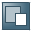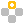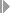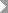Show TOC

###Target Cost Versions in Variance CalculationThe calculation of variances is controlled by a target cost version that you define in Customizing for Product Cost Controlling. In the period-end closing process, you specify which target cost version the system should use to calculate the target costs.

In the standard system you can choose from among the following target cost versions:

• Target cost version 0

Target cost version 0 calculates the total variance.

Target cost version 0 is used to explain the difference between the debit and credit of the product cost collector or manufacturing order. The target costs calculated on the basis of the current standard cost estimate are compared with the actual costs from which the work in process has been deducted. The difference is assigned to different variance categories depending on the cause. Only the variances calculated with target cost version 0 can be transferred to Profitability Analysis (CO-PA).

In target cost version 0 you can specify a valuation variant for work in process and scrap. This valuation variant allows you to control which cost estimate is used to valuate the scrap:

• A preliminary cost estimate for the product cost collector or manufacturing order

• An alternative material cost estimate, such as a modified standard cost estimate for calculation of the planned price of the period

• A current standard cost estimate. In make-to-stock production, this cost estimate is the standard cost estimate for the material. In sales-order-related production with a valuated sales order stock, the cost estimate is selected on the basis of a strategy sequence.

The current standard cost estimate is the cost estimate that is to calculate the standard price in the material master record.

If you don't define a valuation variant for work in process and scrap, the scrap is valuated at the standard costs calculated in the standard cost estimate.

Target cost version 0 can be used in the Product Cost by Period component with cost object hierarchies as a basis for actual cost distribution.

• Target cost version 1

Target cost version 1 calculates the production variances.

Target cost version 1 is used to explain the difference between the target costs calculated on the basis of a preliminary cost estimate for the order and the net actual costs. This allows you to determine the variances that occurred during production. For example, you can determine the variances caused by a changed input quantity and the variances caused by changes in the production structure.

With target cost version 1, production variances for the product cost collector are calculated using the target costs determined on the basis of the preliminary cost estimate for the product cost collector. If you are using a product cost collector, the target costs cannot be determined on the basis of the preliminary cost estimate for the manufacturing order.

Target cost version 1 can be used in the Product Cost by Period component with cost object hierarchies as a basis for actual cost distribution.

Target cost version 1 is used for informational purposes. It cannot be settled.

• Target cost version 2

Target cost version 2 calculates the planning variances.

Target cost version 2 is used to explain the difference between the target costs calculated on the basis of a current standard cost estimate for the material and the planned costs calculated on the basis of a preliminary cost estimate for the manufacturing order. This allows you to determine the variances between the standard cost estimate for the material and the preliminary cost estimate of an order. For example, you can use target cost version 2 to decide whether to manufacture an order with a particular quantity structure.

Target cost version 2 is used for informational purposes. It cannot be settled.

Target cost version 2 cannot be used for product cost collectors. This means that the system cannot calculate variances from the difference between the target costs calculated on the basis of a current standard cost estimate and the preliminary cost estimate for the product cost collector.

• Target cost version 3

Target cost version 3 calculates the production variances of the period.

Target cost version 3 is used to explain the difference between the target costs calculated on the basis of an alternative material cost estimate (such as a modified standard cost estimate) and the net actual costs.Example

Suppose you create a modified standard cost estimate each month. You can use target cost version 3 to calculate the production variances on the basis of your monthly planning.

Target cost version 3 can be used in the Product Cost by Period component with cost object hierarchies as a basis for actual cost distribution.

Target cost version 3 is used for informational purposes. It cannot be settled.

End of the example.Note

Variances are always calculated by comparing the target costs with the control costs.

End of the note.

For information on the calculation of target costs and control costs, refer to the following sections:

Calculation of Target Costs

Calculation of Control Costs

For detailed information on the valuation variant for scrap, refer to the IMG of the Product Cost by Period component and the IMG of the Product Cost by Order component underPeriod-End ClosingVariancesDefine Valuation Variant for Work in Process and Scrap (Target Costs).

For information on the special features of variance calculation and scrap calculation with a valuated sales order stock, refer to the following section:

Variances and Scrap with a Valuated Sales Order Stock

For information on valuation and standard price calculation with a valuated sales order stock, refer to the following sections:

Valuated Sales Order Stock: Valuation

Standard Price with Valuated Sales Order Stock

For information on target cost versions, refer to the IMG for Product Cost by Period and Product Cost by Order underPeriod-End ClosingVariances.

For information on the use of target costs in actual cost distribution, refer to the following sections:

Actual Cost Distribution